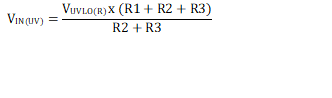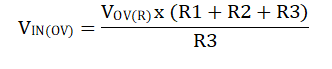SNOSDC0A October   2020  – December 2020

PRODUCTION DATA

1. Features
2. Applications
3. Description
4. Revision History
5. Pin Configuration and Functions
6. Specifications
7. Detailed Description
1. 7.1 Overview
2. 7.2 Functional Block Diagram
3. 7.3 Feature Description
4. 7.4 Device Functional Modes
8. Application and Implementation
1. 8.1 Application Information
2. 8.2 Single Device, Self-Controlled
1. 8.2.1 Typical Application
3. 8.3 Active ORing
4. 8.4 Priority Power MUXing
5. 8.5 USB PD Port Protection
6. 8.6 Parallel Operation
9. Power Supply Recommendations
10. 10Layout
11. 11Device and Documentation Support
12. 12Mechanical, Packaging, and Orderable Information

#### Package Options

• RPW|10
##### 8.2.1.2.1 Setting Undervoltage and Overvoltage Thresholds

The supply undervoltage and overvoltage thresholds are set using the resistors R1, R2 & R3 whose values can be calculated using Equation 6 and Equation 7:

Equation 6.Equation 7.Where VUVLO(R) is the UVLO rising threshold and VOV(R) is the OVLO rising threshold . Because R1, R2 and R3 leak the current from input supply VIN, these resistors must be selected based on the acceptable leakage current from input power supply VIN. The current drawn by R1, R2 and R3 from the power supply is IR123 = VIN / (R1 + R2 + R3). However, leakage currents due to external active components connected to the resistor string can add error to these calculations. So, the resistor string current, IR123 must be chosen to be 20 times greater than the leakage current expected on the EN/UVLO and OVLO pins.

From the device electrical specifications, both the EN/UVLO and OVLO leakage currents are 0.1 μA (max), VOV(R) = 1.2 V and VUVLO(R) = 1.2 V. From design requirements, VIN(OV) = 13.2 V and VIN(UV) = 10.8 V. To solve the equation, first choose the value of R1 = 470 kΩ and use the above equations to solve for R2 = 10.7 kΩ and R3= 48 kΩ.

Using the closest standard 1% resistor values, we get R1 = 470 kΩ, R2 = 11 kΩ, and R3 = 47 kΩ.